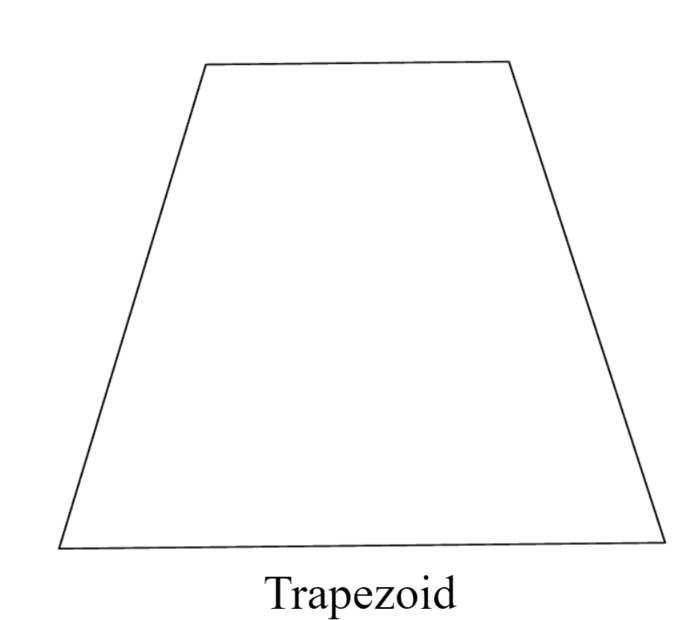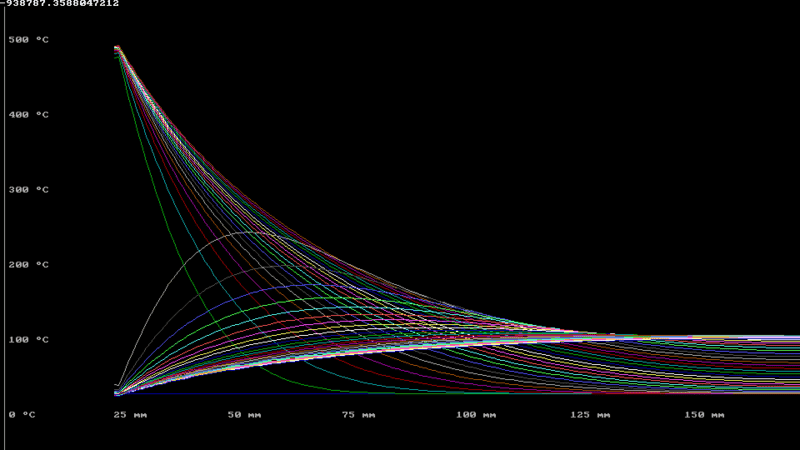# Calculating Transient Heat Transfer in Pipes with Thick Walls

In summary, the heat capacity of concrete can be found online. A heat recovery technique very similar to yours is used to operate old glass-melting furnaces. You might be able to get some empirical data there.

TL;DR Summary
Attempting to calculate the amount of heat able to be stored in large concrete pipes heated via high temperature and pressure air forced through pipes in the concrete.
Hi Everyone,
I am looking to find how much heat can be stored in a concrete pipe of roughly 0.3-0.4m diameter, and an internal diameter of 0.05m. Air will travel through the internal diameter at 500°C and 17.5bar which will provide the heat for the pipes. This system will then be reversed so that cold air is pumped through the system in order to extract the heat.
In terms of output, I want to be able to determine:
• The amount of heat stored in the concrete at given time,
• The time at which heat in vs heat stored becomes inefficient and,
• The temperature distribution through the cross section at a given time.
Due to the thickness of the temperature and the addition of the time variable I believe I should assume unsteady state, and everything I have read about unsteady state heat transfer only seems to in relation to slabs, cylinders, and spheres. I have read a few papers that refer to unsteady state heat transfer in hollow cylinders however the maths really starts to go over my head (see Campos et al.)
What is the best way to go about calculating this? I know I can simulate it using CFD modelling however I was really looking to be able to calculate it in order to generate a table and determine the most effective configurations without running countless simulations.

Cheers,

Baluncore said:
Welcome to PF.
https://www.greenspec.co.uk/building-design/thermal-mass/
Down the page are thermal properties of concrete.
I have the thermal properties of the specific type of concrete that will be used, such as thermal conductivity and specific heat capacity. What I am struggling with is the calculations for the heat transfer as I believe I should assume unsteady state. The concrete exterior will not be the same temperature as the internal temperature at a given time and I just want to calculate this to see what it looks like.

I can't be any specific help with your question, but:

A heat recovery technique very similar to yours is used to operate old (some are still in use) glass-melting furnaces. You might be able to get some empirical data there.

I believe you are looking at a thermal diffusion model.

As you pump heat through the central tube, a wave of rising temperature propagates outwards.
As you draw heat from the central tube, the temperature reduction wave also propagates outwards.

The tube model can be simplified to a short insulated wedge.
That can be modeled in one dimension as a low-pass filter, a ladder with tapered coefficients.

I have a general piece of advice: don't try to solve the hardest problem first. For example, the first thing you might try, just to get your feet wet, is to find the steady-state distribution in the concrete pipe, assuming the hot air is flowing forever at fixed temperature and the outside environment of the pipe is a heat sink that stays at a fixed temperature.

Does the air cool substantially as if flows through the pipe? How long is this pipe? What is the initial temperature before the air flow starts? What is the boundary condition outside the pipe? What is the time scale you are looking at? What is the air mass flow rate?

Last edited:
If you sliced the thick pipe and laid it out flat, you would have a trapezoid shape. The narrow part being the inside surface, and the wide part the outside surface.Analysis of a rectangular slap can approximate that of a trapezoid. Since you already found the equations for the rectangular slab, I recommend starting there. For the next step, consider the ratio of inside surface area to outside surface area.

Reduction of the thick wall pipe to an insulated truncated wedge, quickly converts the problem to a 1D simulation. That has simple analogs.

The volume about the central exchange tube is very quickly heated or cooled, each annular cylinder stores more energy as it has higher volume. Exchange rate between annular cylinders is proportional to reciprocal of surface area of cylinder contact.

Here is a numerical simulation, just to prove how simple it is.
This plot shows radius horizontally, temperature vertically.Blue base line is initial 20°. Then green line is rapid heat of inner to 500°, as temperature wave travels outwards and stores heat in outer region. Then turn off the hot air, to cool with 20° air. White line shows inner temp falls very fast, then draws energy from the outer bulk storage.

Summary:: Attempting to calculate the amount of heat able to be stored in large concrete pipes heated via high temperature and pressure air forced through pipes in the concrete.

Air will travel through the internal diameter at 500°C and 17.5bar
Wondering from where you get, or need, the 17.5 bar pressure.

Sounds like it might be an extensive network of pipes is what is being considered.
What would be the volume mass rate of air, and velocity of the air through a particular pipe.
That can effect the heat transfer for fully developed flow.
Buried underground, which might be a good choice, as the soil between pipes can be used as a heat sink also.
( Insulation of the pipes could end up being a superfluous expense )

Preferred exit temperature of the air - to atmosphere, or recycled?

Are you really going to try to build this?

256bits said:
Buried underground, which might be a good choice, as the soil between pipes can be used as a heat sink also.
( Insulation of the pipes could end up being a superfluous expense )
The system operates between 20°C and 500°C, so the external surface can be expected to average 260°C. If the concrete thermal reservoir is buried and not insulated, that will lead to a continuous loss of heat to the ground environment, causing inefficiency and hot ground, along with underground and surface biological implications.

The time taken for the central flow to extract energy from the outer radius of the concrete tube may be several hours. As a low-pass filter it has an Infinite Impulse Response. While in use it will never reach a steady state.

This high-pressure component could be part of a carbon-capture power plant. Several could be cyclically used as a regenerative heat exchanger with a closed-cycle gas turbine.

•256bits
This OP has not be seen since Tuesday morning, 2 hours after he joined PF. Are we all wasting our time?

•anorlunda
Chestermiller said:
Are we all wasting our time?
No.
I think we have all learned something, with the exception of the OP.

•Haborix
If there is really an array of tubes running through the concrete and the outside of the concrete is insulated, there is a very simple solution for the temperature wave traveling axially through the concrete, that applies in the limit of very high heat transfer coefficient between the air and the concrete.

Chestermiller said:
If there is really an array of tubes running through the concrete and the outside of the concrete is insulated, ...
To keep the model simple there would be an array of concrete cylinders, each insulated from the others, each with one central heat exchange tube.

Because a temperature gradient is required to move the heat, the lowest and highest temperatures would take forever to reach the outer radius. For a 20°C to 500°C core the outer annulus would vary between say 120°C and 400°C. Finding the optimum would be an interesting challenge.

It is the radial wave that has the IIR. If there was a significant axial variation in temperature, then I expect the system should be operated in a tidal mode as a counter-current exchanger.

Chestermiller said:
... there is a very simple solution for the temperature wave traveling axially through the concrete, ...
I would like to know what that is.
But I am definitely concerned with the radial wave of history.

The model is essentially what we use for heat transfer in flow through porous media and granular beds. If ##T_c## is the average temperature of the concrete at a given axial cross section x and ##T_a## is the average temperature of the air at the same cross section, then a heat balance on both the concrete and the air would be: $$\rho_cC_cA(1-\epsilon)\frac{\partial T_c}{dt}=Ua(T_a-T_c)\tag{1}$$$$\dot{m}C_a\frac{\partial T_a}{\partial x}=-Ua(T_a-T_c)\tag{2}$$where ##\rho_c## is the density of the concrete, ##C_c## is the heat capacity of the concrete, A is the total cross sectional area of tubes and concrete, ##\epsilon## is the fraction of the cross sectional area occupied by tubes, U is the overall heat transfer coefficient, a is the heat transfer area per unit length between the tubes and the concrete, ##\dot{m}## is the total mass flow rate of air, and ##C_a## is the heat capacity of the air. Eqn. 2 neglects the accumulation term for the air.

If we add these two equations together, we get:$$\rho_cC_cA(1-\epsilon)\frac{\partial T_c}{dt}+\dot{m}C_a\frac{\partial T_a}{\partial x}=0$$If Ua is very large, then ##T_c## will approach ##T_a## at each cross section, and we will have $$\frac{\partial T}{\partial x}+V_x\frac{\partial T}{\partial x}=0$$where $$V_x=\frac{\dot{m}C_a}{\rho_cC_cA(1-\epsilon)}$$ This will represent the speed of the axial temperature wave through the concrete. Behind the leading edge of the temperature wave, the temperature will be 500 C. Ahead of the temperature wave, the temperature will still be 20 C.

This approximation is only valid in the limit of high Ua. Otherwise there will be axial dispersion ahead of and behind the leading edge of the wave.